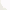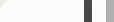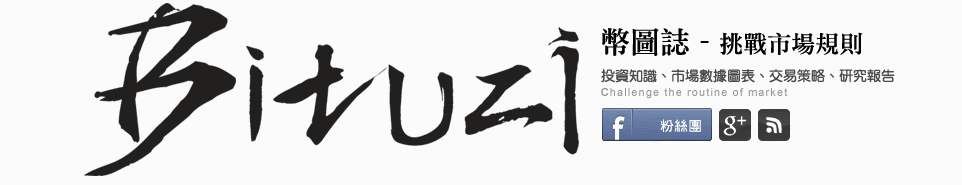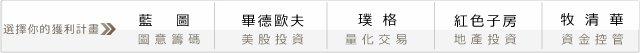## 開發商品的交易系統 - 基礎篇{ BullBeater 指標 }
Input: Length(9), SDev(2), Fct(1.5);
Vars: TBB(0), BBB(0);

TBB = BollingerBand(C,Length, SDev);
BBB = BollingerBand(C, Length, -SDev);

Plot1( TBB - BBB, "BBand Diff" );
Plot2( Average( Range, 4) * Fct, "Min" );Input: Length(9), SDev(2), OSold(45), OBought(55);
Vars: TBB(0), BBB(0), StopPrice(0), TradeTime(false), OS(false), OB(false);

TBB = BollingerBand(Close,Length, SDev);
BBB = BollingerBand(Close, Length, -SDev);
Condition1 = (TBB-BBB) < 1.5*Average(Range,4) OB = RSI(Close,4) > OBought;
OS = RSI(Close,4) < OSold;

if Condition1 and OS then Buy next bar at TBB stop ;

if Condition1 and OB then Sell next bar at BBB stop ;

{ 多單出場 }
if MP = 1 and MP <> 1 then StopPrice = Low - Average(range,4);
If MP = 1 then begin
exitlong next bar at stopprice stop;
stopprice = stopprice + (low-stopprice)/3;
end;

{ 空單出場 }
if MP = -1 and MP <> -1 then
StopPrice = High + Average(range,4);
If MP = -1 then begin
exitshort ("parashort") next bar at stopprice stop;
stopprice = stopprice - (stopprice-high)/3;
end;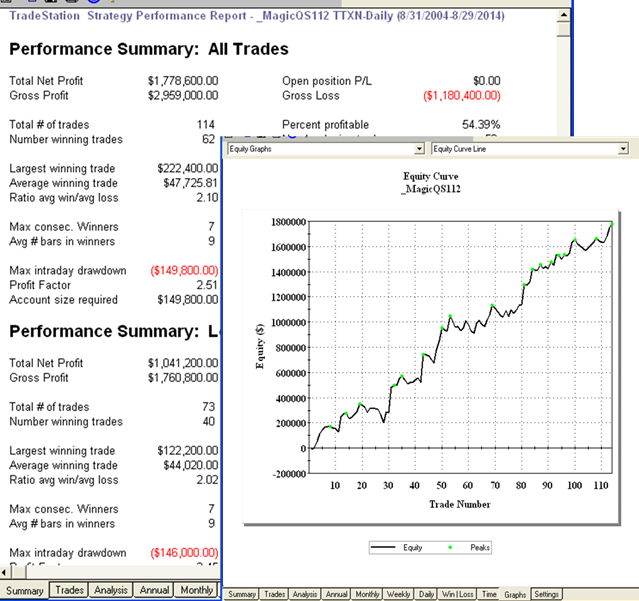#### 0 意見: